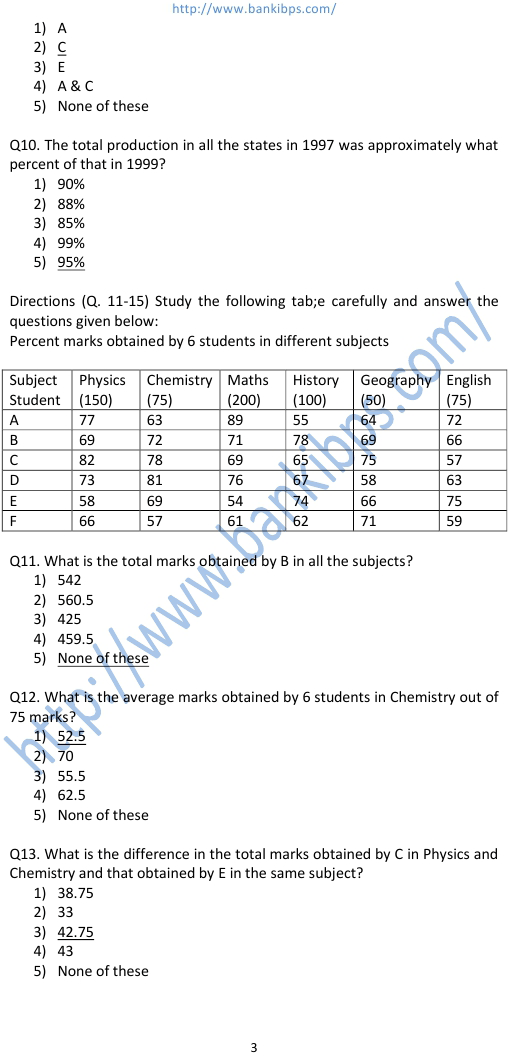# Data Interpretation Question Bank

Q1. What was the strength of Unit B in 1998? 1) 142 2) 125 3) 159 4) 2007 5) None of these Q2. In 1999 the strength of workers was maximum in which unit? 1) E 2) D 3) C 4) B 5) A Q3. The strength of data interpretation question bank workers in unit C in 1996 is approximately what percent of the strength in unit E in 1997? 1) 97 2) 110 3) 104 4) 98 5) 112 Q4. What was the total strength of workers in all the five units in 1996? 1) 647 2) 570 3) 590 4) 697 5) None of these Q5. What was the approximate increase/decrease in the strength of the workers in unit D in 1998 with respect to its initials strength? 1) 47.37% increase 2) 64.46% decrease 3) 64.47% increase 4) 47.37% decrease 5) 59.38% increase
Practice Exercise - 196 [ Data Interpretation ]## data interpretation question bank

### data interpretation questions for bank po

#### data interpretation questions and answers

##### data interpretation questions
###### data interpretation practice
data interpretation questions for bank po. data interpretation questions and answers. data interpretation questions. data interpretation practice. data interpretation questions with answers. sample data interpretation questions. data interpretation test questions. question on data interpretation. data interpretation practice questions. sample questions on data interpretation. data interpretation sample papers. data interpretation questions book. data interpretation download. data interpretation di. data interpretation means. definition of data interpretation. e book data interpretation. data analysis and interpretation questions for bank exams. question of data interpretation with solutions.